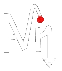#Life Of Navin

## Random Musings, Random Bullshit.### The 23 Toughest Math Problems!!It sounds like a mathophobic's worst nightmare.The folks at the Defense Advanced Research Projects Agency (DARPA) have put out a research request it calls "Mathematical Challenges". The goal of the research is very ambitious: "to dramatically revolutionizing mathematics and thereby strengthening DoD's scientific and technological capabilities."

DARPA is known worldwide for its Urban Grand Challenge competition for Driverless automated cars in urban areas.

So if you're a super-god in math and want to prove it then give one of these a shot.... be warned, these ain't no math puzzles!! What's more, DARPA has stated it will award research grants for the researches but doesn't say how much they'd be worth. So I'm guessing its some astronomically high amount!!

• The Mathematics of the Brain: In my opinion, the toughest math problem ever. the aim is to develop a mathematical theory to build a functional model of the brain that is mathematically consistent and predictive rather than merely biologically inspired.
• The Dynamics of Networks: Develop the high-dimensional mathematics needed to accurately model and predict behavior in large-scale distributed networks that evolve over time occurring in communication, biology and the social sciences.
• Capture and Harness Stochasticity in Nature: Address Mumford's call for new mathematics for the 21st century. Develop methods that capture persistence in stochastic environments.
• 21st Century Fluids: Classical fluid dynamics and the Navier-Stokes Equation were extraordinarily successful in obtaining quantitative understanding of shock waves, turbulence and solitons, but new methods are needed to tackle complex fluids such as foams, suspensions, gels and liquid crystals.
• Biological Quantum Field Theory: Quantum and statistical methods have had great success modeling virus evolution. Can such techniques be used to model more complex systems such as bacteria? Can these techniques be used to control pathogen evolution?
• Computational Duality: Duality in mathematics has been a profound tool for theoretical understanding. Can it be extended to develop principled computational techniques where duality and geometry are the basis for novel algorithms?
• Occam's Razor in Many Dimensions: As data collection increases can we "do more with less" by finding lower bounds for sensing complexity in systems? This is related to questions about entropy maximization algorithms.
• Beyond Convex Optimization: Can linear algebra be replaced by algebraic geometry in a systematic way?
• What are the Physical Consequences of Perelman's Proof of Thurston's Geometrization Theorem?: Can profound theoretical advances in understanding three dimensions be applied to construct and manipulate structures across scales to fabricate novel materials?
• Algorithmic Origami and Biology: Build a stronger mathematical theory for isometric and rigid embedding that can give insight into protein folding.
• Optimal Nanostructures: Develop new mathematics for constructing optimal globally symmetric structures by following simple local rules via the process of nanoscale self-assembly.
• The Mathematics of Quantum Computing, Algorithms, and Entanglement: In the last century we learned how quantum phenomena shape our world. In the coming century we need to develop the mathematics required to control the quantum world. Something I'll dedicate my life to studying in the future.
• Creating a Game Theory that Scales: What new scalable mathematics is needed to replace the traditional Partial Differential Equations (PDE) approach to differential games?
• An Information Theory for Virus Evolution: Can Shannon's theory shed light on this fundamental area of biology?
• The Geometry of Genome Space: What notion of distance is needed to incorporate biological utility?
• What are the Symmetries and Action Principles for Biology?: Extend our understanding of symmetries and action principles in biology along the lines of classical thermodynamics, to include important biological concepts such as robustness, modularity, evolvability and variability.
• Geometric Langlands and Quantum Physics: How does the Langlands program, which originated in number theory and representation theory, explain the fundamental symmetries of physics? And vice versa?
• Arithmetic Langlands, Topology, and Geometry: What is the role of homotopy theory in the classical, geometric, and quantum Langlands programs?
• Settle the Riemann Hypothesis: The Holy Grail of number theory. Do read about it!!
• Computation at Scale: How can we develop asymptotics for a world with massively many degrees of freedom?
• Settle the Hodge Conjecture: This conjecture in algebraic geometry is a metaphor for transforming transcendental computations into algebraic ones.
• Settle the Smooth Poincare Conjecture in Dimension 4: What are the implications for space-time and cosmology?? And might the answer unlock the secret of "dark energy"??
• What are the Fundamental Laws of Biology?: This question will remain front and center for the next 100 years. DARPA places this challenge last as finding these laws will undoubtedly require the mathematics developed in answering several of the questions listed above.
You need to submit your research plan by Sept. 29, 2009. So get going people!!

Anonymous said...

Hey Navin, nice to see tht Reinmann hypothesis on the list!

U know how much I talk about it

Great mystery

Definitely my pick for toughest math problem ever

Anonymous said...

yeah I agree with satish

a lot of math works simply because it assumes the Reinmann hypothesis to be correct.

if its proved wrong, then mathematics as we know it would collapse